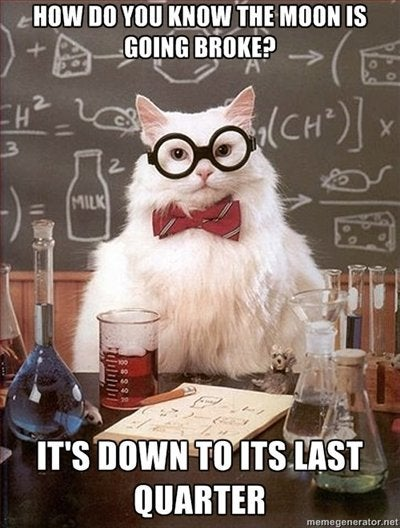Science

# These 'X-Rated' Math Terms Sound Dirty But Aren't Really

For some people, math is a four-letter word. But even if you're comfortable talking equations, you might be a bit squeamish when making reference to math terms with names that sound X-rated.

Here are six math terms that sound dirty but aren't (see list below). The explanations for the terms were created with help from Steven H. Strogatz, a professor of mathematics at Cornell University and the author of a popular book about math whose own title sounds a bit racy, "The Joy of X: A Guided Tour of Math, From One to Infinity."

Cox-Zucker machine. What sounds like a high-tech device for oral sex is actually an algorithm used in the study of certain curves, including those that arise in cryptography. The story goes that David A. Cox co-authored a paper with fellow mathematician Steven Zucker, just so that the dirty-sounding term would enter the lexicon.

Fuzzy set. An ordinary set is like a club -- you're in or you're out. With a fuzzy set, membership is a bit uncertain. Something can be both partially inside the set and partially outside. Fuzzy sets can be useful in biology and engineering in situations in which information is incomplete or imprecise.

Hairy ball theorem. This theorem is about how vectors can be arrayed on the surface of a sphere. Imagine a sphere covered with fur -- with each strand of hair representing a vector. According to the hairy ball theorem, it's impossible to comb the hair without creating a cowlick somewhere on the sphere.

LaTeX. Not for rubber fetishists. LaTeX is actually an offshoot of TeX (pronounced "tech," a reference to technical typing), a popular computer package for typesetting mathematical terms and equations. LaTeX, which is considered more user-friendly than TeX, was named for its developer, American computer scientist Leslie Lamport, 73.

Tits alternative. No, it's not about alternatives to breasts. The Tits alternative is a theorem discovered by French mathematician Jacques Tits, 83. It states that a certain class of mathematical groups has either one type of internal structure or another -- that's the alternative. Group theory has proven helpful in the study of everything from particle physics to Rubik's cubes as well as mathematical equations.

Wiener measure. Size matters in math too. But the Wiener measure, named after American mathematician Norbert Wiener, who died in 1964, is an indication of how likely it is for a continuous function (think of a graph showing daily fluctuations in stock prices) to lie within certain limits.27 Funniest, Geekiest Science Jokes